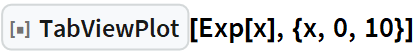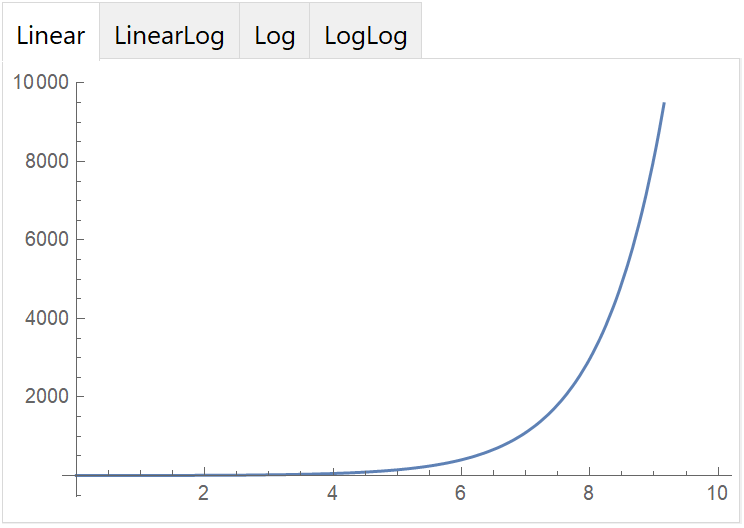# Wolfram CloudResource Object

Function Resource

# TabViewPlot

Create a TabView for a Plot using linear and logarithmic axes

 ResourceFunction["TabViewPlot"][args] creates a TabView for plots using linear and logarithmic axes.

## Details and Options

The TabView has four tabs:
 Linear both axes are linear LinearLog x-axis is logarithmic Log y-axis is logarithmic LogLog both axes are logarithmic

## Examples

### Basic Examples

Visualize ex using linear and logarithmic axes:

 In:=Out=